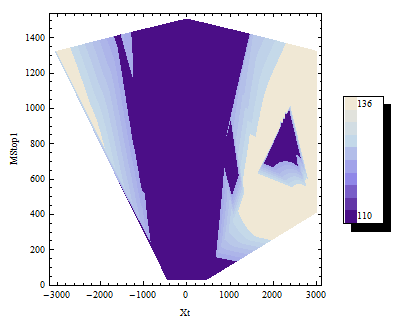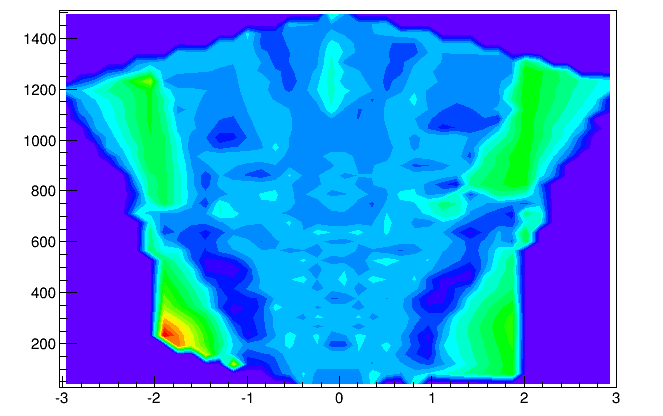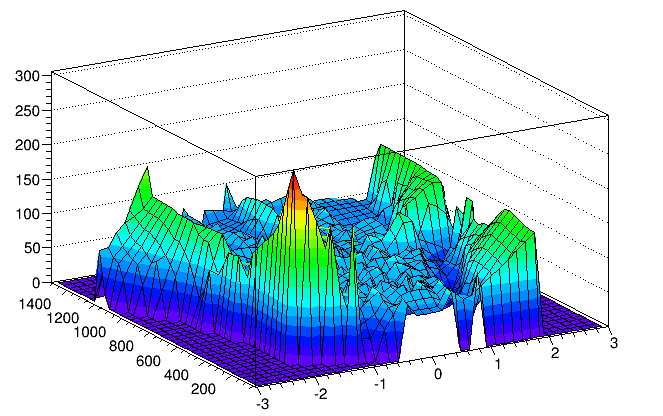# Help importing and plotting 3D data

Greetings!

I just started using ROOT and even though I spent several days reading, I do not understand why my simple code is not working…

I have a test file containing many columns of data, which I import using ifstream and select three of them to plot using TGraph2D. The data is imported and the numbers are correct. I used two methods (plot.cxx and plot3.cxx, which as expected gives the same result as plot2.cxx) to set the points.
The first one (plot.cxx) just spits out the error:

``Two of these three points are coincident blah blah``

and causes ROOT to go into an infinite loop (it just keeps printing the same error about the same data point over and over and I have to kill ROOT).
The second one does not give any error, but the graph is empty (like all z-values are 0), even though the axis ranges are set automatically to the correct values (min and max values in my data).
I do not understand where I’m going wrong.

P.S. I tried importing the data using TTree as was done in the tree/basic.C tutorial, but this gives the following error for every single line of the data:

``Couldn't read formatted data in....``

Even if I run the tutorial itself (basic.C) it gives the same thing instead of do what it’s supposed to! Am I supposed to set some env variable to make this work??
plot3.cxx (948 Bytes)
plot2.cxx (1019 Bytes)
plot.cxx (665 Bytes)

Hello, I looked at quickly your codes and in plot.cxx, did you try to put “i++” just after

Normally, pl is expecting to have a point at “0”.
For plot2 and plot3, did you try to use directly the vector to “construct” your TGraph2D ; something like that

Normally, it is exactly the same as what you did with the arrays.

Finally, I guess, you have check that your vector does not contain only empty values.

EDIT : I modified a little bit your plot2.cxx and I got a running example

[code]#include

using namespace std;

int test()
{
TCanvas *c_pl = new TCanvas(“c_pl”);
vector < float > v_xt, v_mst1, v_mh1;

for(int i=0 ; i<10 ; i++) {
v_xt.push_back(i);
v_mst1.push_back(pow(i,i));
v_mh1.push_back(i*i);
}

const unsigned int n = v_xt.size();
Float_t a_xt[n], a_mst1[n], a_mh1[n];
for (Int_t i = 0 ; i < n ; i++)
{
a_xt[i] = v_xt.at(i);
a_mst1[i] = v_mst1.at(i);
a_mh1[i] = v_mh1.at(i);
}
TGraph2D * pl = new TGraph2D(n, a_xt, a_mst1, a_mh1);
pl->Draw(“SURF1”);
return 0;
}[/code]
You can run it doing “.x test.C”

[quote=“pamputt”]Hello, I looked at quickly your codes and in plot.cxx, did you try to put “i++” just after

``pl->SetPoint(i,xt,mst1,mh1);``

Normally, pl is expecting to have a point at “0”.
[/quote]
Hi and thanks for replying! I didn’t know that, I thought its point numbers start at 1, that’s why I had done it like that. Using your suggestion does indeed fix the issue with the errors and the loop and now all three .cxx files give the same (flat) graph.

I tried that now - same result.

I had done that in the beginning while debugging and none of them are zero - all three coordinates lie in the ranges they should (I outputted all of them)…

I exported my data (not using ROOT), this time selecting only these three columns and these ranges, so that I can attach the file (otherwise it’s HUGE). So there they are, but still it gives just a flat graph…

Edit:
I had made a mistake in plots.cxx (recalculating xt from values I wasn’t even importing). I fixed it (the file is now updated), but still flat graph.
plot_selected.cxx (368 Bytes)
data_selected.dat (69.3 KB)

Hmm, I do not know really TGraph2D and its Draw option. Anyway, I got also a flat graph with the “surf” option. But if I use “p” option (instead of surf), I got something consistent with your data. Same result with other options shown here
So you have to investigate this “surf” option. I have no ideaTips : to fill your TGraph2D, you can skip the “ifstream step” by doing simply

```{ TGraph2D * pl = new TGraph2D("data_selected.dat","%lf%lf%lf"); pl->Draw("pcol"); }```

[quote=“pamputt”]Hmm, I do not know really TGraph2D and its Draw option. Anyway, I got also a flat graph with the “surf” option. But if I use “p” option (instead of surf), I got something consistent with your data. Same result with other options shown here
So you have to investigate this “surf” option. I have no idea[/quote]
True, this way I get my plot, but not really in the form I need itI need it to be of the form plt1xth1.png (this is actually the same data but plotted in Mathematica and in the full range). I saw [url=https://root-forum.cern.ch/t/tgraph2d-and-contour-plots/14372/1 topic and thought that the option COL, CONT4 or CONT5 used there would work, but they all give a blank 2D surface…

[quote=“pamputt”]
Tips : to fill your TGraph2D, you can skip the “ifstream step” by doing simply

```{ TGraph2D * pl = new TGraph2D("data_selected.dat","%lf%lf%lf"); pl->Draw("pcol"); }```[/quote]
Thanks for the tip! Can I make this work with my full data set (18 columns) and choose two of them as y and z and calculate x from other columns?I figured out what was wrong! My z function wasn’t single-valued (it had a small dependency on other parameters as well, which were not plotted). Mathematica didn’t have a problem, because it simply took the first point with coordinates x and y and ignored all subsequent points with the same x and y coordinates. However ROOT ignored ALL points which were ambiguous (when drawing surface or contours). I edited my data to remove such points and I got my plots! Thanks for the help again!Thanks! I was planning to change the colour palette anyway, but of course first I had to get something plotted instead of blank plot.

of course